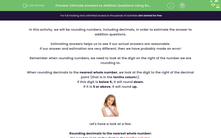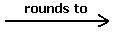### Comprehensive & curriculum aligned

In this worksheet, students will round numbers in order to estimate the answer to addition questions.Key stage:  KS 2

Curriculum topic:   Number: Addition, Subtraction, Multiplication and Division

Curriculum subtopic:   Use Estimation to Check Answers

Difficulty level:#### Worksheet Overview

In this activity, we will be rounding numbers, including decimals, in order to estimate the answer to addition questions.

Estimating answers helps us to see if our actual answers are reasonable.

If our answer and estimation are very different, then we have probably made an error!

Remember when rounding numbers, we need to look at the digit on the right of the number we are rounding to.

When rounding decimals to the nearest whole number, we look at the digit to the right of the decimal point (that is in the tenths column).

If this digit is below 5, it will round down.

If it is 5 or above, it will round up.Let's have a look at a few:

Rounding decimals to the nearest whole number:

We need to look at the digit in the tenths column.

34.2 will round down to 34 (there is a 2 in the tenths column), but 34.6 will round up to 35 (there is a 6 in the tenths column).

43.28 will round down to 43 (there is a 2 in the tenths column), but 43.68 will round up to 44 (there is a 6 in the tenths column).

Rounding to the nearest 10:

We need to look at the digit in the ones column.

43 will round down to 40 (there is a 3 in the ones column), but 45 will round up to 50 (there is a 5 in the ones column).

Rounding to the nearest 100:

We need to look at the digit in the tens column.

538 will round down to 500 (there is a 3 in the tens column), but 578 will round up to 600 (there is a 7 in the tens column).Example

Estimate the answer to the addition by first rounding both decimals to the nearest whole numbers.

3.2 + 5.7A
+
 B
=
 C

3.2 + 5.73
+
 6
=
 9

The estimation of 9 shows that 8.9 is probably the correct answer.

Let's do another:

Estimate the answer to the addition by first rounding both to the nearest ten.

68 + 24A
+
 B
=
 C

68 + 2470
+
 20
=
 90

The estimation of 90 shows that 92 is probably the correct answer.

Now, it's your turn to have a go at some questions.

### What is EdPlace?

We're your National Curriculum aligned online education content provider helping each child succeed in English, maths and science from year 1 to GCSE. With an EdPlace account you’ll be able to track and measure progress, helping each child achieve their best. We build confidence and attainment by personalising each child’s learning at a level that suits them.

Get started••••